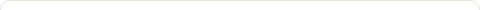# Einstein speaks on: Origin of General Relativity

Posted on: November 21, 2009

Einstein speaks his mind processes on the origin of General Relativity; (Nov. 21, 2009)

This article is on  how Einstein described his mind processes that lead to the theory of restricted relativity and then his concept for General Relativity. In 1905, restricted relativity discovered the equivalence of all systems of inertia for formulating physics equations.

From a cinematic perspective, there was no way to doubting relative movements. Still, there was the tendency among physicists to physically extend privileged significance to system of inertia.  The question was “if speed is relative then, do we have to consider acceleration as absolute?”

Ernest Mach considered that inertia did not resist acceleration except when related to the acceleration toward other masses. This idea impressed Einstein greatly.  Einstein said: ” First, I had to establish a law of gravitation field and suppress the concept of absolute simultaneity. Simplicity urged me to maintain Laplace’s “scalar gravity potential” and fine tune Poisson’s equation.

Given the theorem of inertia of energy then, inertia mass must be depended on gravitation potential; but my research left me skeptical. In classical mechanics, vertical acceleration in a vertical field of gravity is independent of the horizontal component of velocity; it follows that vertical acceleration is exercised independently of the internal kinetic energy of the body in movement.

I discovered that this independence did not exist in my draft theory; this evidence did not coincide with the affirmation that all bodies submit to the same acceleration in a gravitational field. Thus, the principle that there is equality between inertia mass and weight grew with striking significance. I was convinced of its validity, though I had no knowledge of the results of experiments done by Eotvos.”

Consequently, the principle of equality between inertia mass and weight would be explained as follows: in a homogeneous gravitational field, all movements are executed in relation to a system of coordinates accelerating uniformly as if in absence of gravity field. I conjectured that if this principle is applicable to any other events then it can be applied to system of coordinates not accelerating uniformly.

These reflections occupied me from 1908 to 1911 and I figured that the principle of relativity needed to be extended (equations should retain their forms in non uniform accelerations of coordinates) in order to account for a rational theory of gravitation; the physical explanation of coordinates (measured by rules and clocks) has to go.

I reasoned that if in reality “a field of gravitation used in system of inertia” did not exist it could still be served in the Galilean expression that “a material point in a 4-dimensional space is represented by the shortest straight line”. Minkowski has demonstrated that this metric of the square of the distance of the line is a function of the squares of the differential coordinates.  If I introduced other coordinates by non linear transformation then the distance of the line stay homogeneous if coefficients dependent on coordinates are added to the metric (this is the Riemann metric in 4-dimension space not submitted to any gravity field). Thus, the coefficients describe the field of gravity in the selected system of coordinates; the physical significance is just related to the Riemannian metric. This dilemma was resolved in 1912.

Two other problems had to be resolved from 1912 to 1914 with the collaboration of Marcel Grossmann.

The first problem is stated as follows: “How can we transfer to a Riemannian metric a field law expressed in the language of restrained relativity?”  I discovered that Ricci and Levi-Civia had answered it using infinitesimal differential calculus.

The second problem is: “what are the differential laws that determine the coefficients of Riemann?”  I needed to resolve invariant differential forms of the second order of Riemann’s coefficients. It turned out that Riemann had also answered the problem using curb tensors.

“Two years before the publication of my theory on General Relativity” said Einstein “I thought that my equations could not be confirmed by experiments. I was convinced that an invariant law of gravitation relative to any transformations of coordinates was not compatible with the causality principle. Astronomic experiments proved me right in 1915.”

Note:  I recall that during my last year in high school my physics teacher, an old Jesuit Brother, filled the blackboard with partial derivatives of Newton’s equation on the force applied to a mass; then he integrated and he got Einstein’s equation of energy which is  mass multiplied by C square. At university, whenever I had problems to solve in classical mechanics on energy or momentum conservation I just applied the relativity equation for easy and quick results; pretty straightforward; not like the huge pain of describing or analyzing movements of an object in coordinate space.

### 1 Response to "Einstein speaks on: Origin of General Relativity"space and time. relativity and light speed. gravity the force how many Gssssssssss that Astronauts are able to with stand going up in space many different ways how gravity is used like flying in a military jet plain . all kinds of ways how the use of gravity is being used I feel we will do the same of many people that has come to visit us those that know how free energy works.### Blog Stats

• 1,429,109 hits